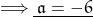f(x) = 3x + 5 Work out a if f(a)= -13

Question

f(x) = 3x + 5
Work out a if f(a)= -13

in progress 0
6 months 2021-07-19T22:09:03+00:00 2 Answers 4 views 0

a = -6

Step-by-step explanation:

see, x has been replaced by a.

so f(a) = 3a + 5 . . . . . . . (i)

a displaces x from it’s every position in the equation.

and it’s given that f(a) = -13

putting f(a) = -13 in equation (i) :-

3a + 5 = -13

• taking like terms to one side

3a = -18

• dividing the equation by 3a = – 6

Step-by-step explanation:

Given

f(x) = 3x + 5 , then

f(a) = 3a + 5 and f(a) = – 13 , then equating the right sides

3a + 5 = – 13 ( subtract 5 from both sides )

3a = – 18 ( divide both sides by 3 )

a = – 6